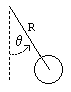# Chaotisches Pendel

vorherig nächste
• Sim
• Graph
• Zeit Graph
• MehrGraphen

A damped driven pendulum is a chaotic system. In a chaotic system the future behavior is highly dependent on the exact value of the initial conditions. A tiny change in initial conditions can cause huge changes after a short period of time.

Click the "Sim" tab to access parameters such as: drive amplitude, frequency, mass, gravity, and damping. You can drag the pendulum with your mouse to change the starting position.

The buttons below set parameters so the simulation is either in a loop or in a chaotic state; the drive amplitude is different in each case and shown in parenthesis. It takes around a minute to settle into a loop.

The math behind the simulation is shown below. Also available are: open source code, documentation and a simple-compiled version which is more customizable.

## The Damped Driven Pendulum

The pendulum is subject to frictional damping, meaning that it will slow down over time if there is no driving force. To see this try setting the drive amplitude to zero, so the only forces are damping and gravity.

The driving force is shown as a curved arrow in the simulation. The length of the arrow corresponds to the amount of torque (twisting force) at a given moment. The direction of the arrow, clockwise or counter-clockwise, shows the direction of this applied force. You can change the amplitude (strength) of the driving force or its frequency (how often it switches directions).

Not all combinations of the parameters (length, gravity, drive amplitude, drive frequency, damping) will lead to chaos. Many combinations result in simpler repeating behavior. Click the "single loop" button above to see this. You'll need to wait about a minute for the simulation to settle into the loop.

There is a phenomenon known as period doubling or bifurcation where slowly increasing one of the parameters, such as drive amplitude, causes the number of loops in the repeating behavior to double. Some of the above buttons change the drive amplitude gradually so that you see this period doubling occur. For example, you get a single loop with drive amplitude of 1.35, a double loop with 1.45, a quadruple loop with 1.47, and eventually you get chaos at 1.50.

After enough period doublings occur, the system becomes chaotic. Click one of the buttons labelled "chaos" to set the parameters and see this. A curious thing about this chaotic behavior is that it is not entirely random. While you can't predict the exact state of the system at a given time in the future, it is possible to show that the system will follow an elaborate pattern, These patterns are fractals, which are patterns that repeat themselves when you magnify them.

## Physicspendulum variables

The pendulum is modeled as a point mass at the end of a massless rod. The damping (friction) is proportional to the angular velocity of the pendulum. There is also an external driving force which provides a periodic torque (twist). Define the following variables:

• θ = angle of pendulum ( 0 = vertical)
• ω = θ' = angular velocity
• R = length of rod
• m = mass of pendulum
• g = gravitational constant
• b = damping (friction) constant
• A = amplitude of driving force
• k = constant related to frequency of driving force
• t = time in seconds

We will derive the equation of motion for the pendulum using the rotational analog of Newton's second law for motion about a fixed axis, which is

 I α = ∑ τ (1)
• τ = sum of applied torques
• I = rotational inertia
• α = θ'' = angular acceleration

The rotational inertia about the pivot is I = m R2 . Torque can be calculated as the vector cross product of the position vector and the force.

• torque due to gravity is τ = −R m g sin θ .
• torque due to friction is τ = −b ω .
• torque due to driving force is τ = A cos(k t) .

So equation (1) becomes

m R2 α = −R m g sin θb ω + A cos(k t)

which we can write as

 θ'' = − g⁄R  sin θ + −b θ' + A cos(k t) m R2

This is the equation of motion for the driven damped pendulum.

## Numerical Solution

To solve the equation of motion numerically, so that we can run the simulation, we use the Runge Kutta method for solving sets of ordinary differential equations. First we define a variable for the angular velocity ω = θ' . We also add an equation for time because time appears explicitly in the equations in the driving force A cos(k t) . Then we can write the second order equation above as three first order equations.

t' = 1

θ' = ω

 ω' = − g⁄R sin θ + −b ω + A cos(k t) m R2

This is the form needed for using the Runge-Kutta algorithm.

This web page was first published September 2001.

vorherig nächste